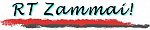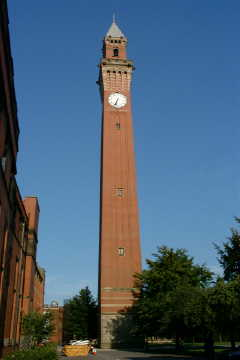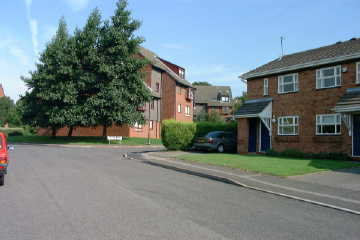Sitemap | Coverstory | Photos | Railways z. | Bridges z. | Seeds
\$B8=:_\$N%Z!<%8(B :
 \$B%H%C%W(B >> \$B%P!<%_%s%,%`JX\$j(B >> 2001\$BG/(B >> 7-9\$B7n(B >> \$B%G%8%+%aJX\$j(B \$BBh(B1\$B2s(B
You are now in :
 Top >> From Birmingham >> Yr. 2001 >> 3rd Q. >> Digital Camera Report No. 1

\$B%P!<%_%s%,%`!&%G%8%+%aJX\$j(B
Digital Camera Report from Birmingham

# \$BBh(B1\$B2s(B \$B!VBgN&4V\$*0z1[\$7!W(BNo. 1: "Intercontinental removals"

 After moving from Japan to UK, I now understand the reality behind an episode from "TNG" (Star Trek: The Next Generation) entitled "The Inner Light". In this episode, a space probe from a planet destroyed more than a thousand years ago delivers the memory of the life there. Indeed, it was done in such a high-tech way, and at first sight I thought: "Why didn't they escape instead of doing all these?" Probably the staff engaged in the creation of the episode had a personal experience of intercontinental movement. Yes, I had to leave many things in Japan because of the limitation in transport! Very fortunately, I was appointed by the University of Birmingham after an interesting selection process. After the exchange of some e-mails, I downloaded an application form in Microsoft Word file format from University web site, filled it in and returned by airmail; before the interview I was asked to send Microsoft PowerPoint data to the selection committee; and, the interview was done over the phone line, looking at the same PowerPoint data on both sides. So, now the information can now easily travel at light speed; small things like documents can be sent very quickly thanks to the improved services using aircrafts. However, there is one thing, without weight, but can hardly be transferred across the border... my own credit. The letter of my supervisor, Dr C J Goodman, was necessary whenever I open bank account or get a place to live in. Telephone and electric utility companies request deposits. The issuing of credit cards were refused due to the "credit scores", even though I have got "Gold Cards" which was issued back in Japan!

## \$B>pJs\$O(B light speed \$B\$G!D!D\$G\$b(B

\$B%"%a%j%+\$N(BSF\$B%F%l%S%I%i%^(B \$B!V?7%9%?!<%H%l%C%/!W(B (Star Trek: The Next Generation, TNG) \$B\$K!"(B The Inner Light \$B\$H\$\$\$&%(%T%=!<%I(B \$B!JK.Bj\$O\$d\$d0B\$C\$]\$/!VD6;~6uOG@1%+%?!<%s!W!K(B \$B\$,\$"\$k!#(B \$B@iG/0J>eA0\$KLG\$s\$@@1\$+\$i!"(B \$B\$=\$N@1\$NJk\$i\$7\$N5-21\$r>h\$;\$?C5::5!\$,\$d\$C\$F\$-\$F!"(B \$B1'ChA%%(%s%?!<%W%i%\$%:\$N4OD9\$K\$=\$N5-21\$rEA\$(\$k!"(B \$B\$H\$\$\$&FbMF\$G!"(B \$B\$9\$0\$l\$?(BSF\$B:nIJ\$KB#\$i\$l\$k(B \$B!V%R%e!<%4!<>^!W(B \$B\$N(B1993\$BG/EY:GM%=(1GA|:nIJ>^\$r^\$7\$?\$=\$&\$@!#(B

\$B\$1\$l\$I!"(B \$B:G6a(BTNG\$B\$K(B \$B!V%O%^%C\$F!W(B \$B\$\$\$k\$i\$7\$\$M'?M\$K\$3\$N%(%T%=!<%I\$r8+\$;\$F\$b\$i\$C\$?\$H\$-(B \$B!V\$I\$&\$7\$F\$=\$N@1\$N?M\$OLG\$S\$kA0\$KF(\$2=P\$5\$J\$+\$C\$?\$N\$+!W(B \$B\$H;W\$C\$?!#(B \$B2?\$7\$m!"(B \$B\$=\$N5-21\$r4OD9\$KEA\$(\$kJ}K!\$,!"(B \$B2?\$H\$+%S!<%`\$G4OD9\$N0U<1\$r>h\$C \$B:#2s!"(B \$B \$B\$?\$@\$NN99T\$@\$C\$?\$i!"(B \$B@.ED\$+\$i%m%s%I%s\$^\$GD>9TJX\$G(B12\$B;~4V\$A\$g\$C\$H!#(B \$B%9!<%D%1!<%9\$R\$H\$D\$"\$l\$P==J,\$@\$m\$&!#(B \$B\$7\$+\$7!"(B \$B0z1[\$7\$N>l9g\$O@83h\$r\$"\$kDxEYJz\$(\$F\$\$\$/I,MW\$,\$"\$k!#(B \$BKM\$N>l9g!"(B \$BBgNL\$N;qNA\$r;}\$A9~\$`\$3\$H\$K\$7\$?\$N\$,!"(B \$BFC\$K\$\$\$1\$J\$+\$C\$?\$+\$bCN\$l\$J\$\$(B \$B!JK\;o\$J\$I!"(B \$B\$J\$s\$HAO4)9f\$+\$i\$9\$Y\$FAw\$C\$?\$N\$@!*!K!#(B \$BA%JX\$G(B1m3\$B!"(B \$B9R6uJX\$G(B25kg\$B!_(B1\$BH"\$N2YJ*\$rJLAw!#(B \$B\$5\$i\$K!"(B \$B8BEY\$.\$j\$.\$j(B \$B!J TNG \$B\$O%"%a%j%+\$N%I%i%^\$@\$+\$i!"(B \$BBgN&4V0\=;\$N7P83\$r;}\$D%9%?%C%U\$,B?\$=\$&\$@!#(B \$B\$3\$N%(%T%=!<%I\$b!"(B \$BH`\$i\$N8D?ME*7P83\$NH?1G\$@\$C\$?\$N\$+\$bCN\$l\$J\$\$!#(B \$BpJs\$O(B light speed \$B\$GAw\$l\$F\$b!"(B \$B2YJ*\$O\$=\$&\$O\$\$\$+\$J\$\$\$N\$@!#(B[Photo-1] \$BBg3X\$NCf?4\$K\$"\$k(B Chamberlain Clock Tower\$B!#D.\$N\$"\$A\$3\$A\$+\$i\$h\$/\$_\$(\$k!#(B \$B!J(B2001. 8. 14 \$B;#1F!#(B\$BBg\$-\$J2hA|(B(JPEG): 70kB\$B!K(B Chamberlain Clock Tower in the middle of the campus, which can be seen from many parts of the area around. (Photo taken: 14 Aug 2001. Larger image (JPEG): 70kB)

## \$B9q:]EEOC\$G(B interview

\$BKM\$O!"(B \$BEl5~Bg3X\$HEl5~EENO\$G\$=\$l\$>\$l(B3\$BG/\$:\$D!"(B \$B9g7W(B6\$BG/4V\$K\$o\$?\$C\$F!"(B \$B9qFb\$G8&5f6HL3\$K=>;v\$7\$F\$-\$?!#(B \$B\$=\$N8e!"(B 2001\$BG/(B7\$B7n\$+\$i!"(B \$B1o\$"\$C\$F1Q9q(B The University of Birmingham \$B!J%P!<%_%s%,%`Bg3X!K(B \$B\$N(B Colin J. Goodman \$B@h@8(B \$B!J@lLg\$OEE5\$E4F;!K(B \$B\$N8&5f%0%k!<%W\$G8&5f0w\$H\$7\$F6PL3\$9\$k\$3\$H\$K\$J\$j!"(B Birmingham \$B\$K0\\$C\$F\$-\$?!#(B

\$B\$3\$N5!2q\$rF@\$k\$K\$"\$?\$C\$F\$O!"(B \$BKM\$b8xJg\$KBP\$7\$F1~Jg=qN`\$rDs=P\$7!"(B \$BA*9M\$r7P\$F:NMQ\$5\$l\$?!#(B \$B\$=\$N8xJg9-9p\$,\$^\$:=P\$k>l=j\$O!"(B \$B\$\$\$^\$d%\$%s%?!<%M%C%H\$N%&%'%V%Z!<%8\$G\$"\$k!#(B \$B1~Jg=qN`\$N=q<0\$b(B Microsoft Word \$BJ8=q%U%!%\$%k\$N7A<0\$G8x3+\$5\$l\$F\$\$\$k\$+\$i!"(B \$B%@%&%s%m!<%I\$7\$F:n@.\$7!"(B \$BJVAw\$9\$l\$P\$h\$\$!#(B \$B!J\$5\$9\$,\$KEE;R%a!<%k\$O\$@\$a\$@\$,!K(B

\$B0lO"\$N7P0^\$N\$J\$+\$G\$b!"(B \$BLL@\(B (interview) \$B\$,\$b\$C\$H\$b6C\$/\$Y\$-%9%?%\$%k\$@\$C\$?\$m\$&!#(B Goodman \$B@h@8\$N\$4Ds0F\$K\$h\$C\$F9T\$o\$l\$?EEOC(B interview \$B\$O!"(B \$BM=\$a(B Microsoft PowerPoint \$B\$N%W%l%<%s%F!<%7%g%s%G!<%?\$rF|K\\$+\$iEE;R%a!<%k\$GAw?.\$7\$F\$*\$-!"(B \$B\$=\$N%G!<%?\$rF|1Q\$GF1;~\$KD/\$a\$D\$D!"(B \$B%W%l%<%s%F!<%7%g%s\$H[Photo-2] \$BI. \$B!J(B2001. 8. 15 \$B;#1F!#(B\$BBg\$-\$J2hA|(B(JPEG): 110kB\$B!K(B The area around my flat. Only 20 minutes' walk from the laboratory. (Photo taken: 15 Aug 2001. Larger image (JPEG): 110kB)

## \$B?.MQ\$OAw?.\$G\$-\$J\$\$!)(B

\$B:NMQ7hDj8e!"(B \$BBg3XEv6I\$,!"(B \$B1Q9q8[MQ>J\$KBP\$7\$FKM\$NO+F/5v2D>Z\$N?=@A\$r\$9\$k!#(B \$B;~4V\$,\$+\$+\$k\$H\$\$\$m\$s\$J?M\$K6<\$5\$l\$?\$,!"(B \$B\$3\$l\$bF|K\\$+\$i\$N=qN`E~Ce8e\$o\$:\$+(B1\$B=54V\$[\$I\$G8rIU\$5\$l\$?!#(B \$BBg3X\$d8[MQ>J\$N;vL3=hM}\$,?WB.\$K\$J\$C\$?\$3\$H\$b\$"\$k\$,!"(B \$B\$d\$O\$j9R6u5!\$r@8\$+\$7\$?9bB.M9JXEy\$N%5!<%S%9\$,=< \$B\$@\$,!"(B \$B=qN`\$,B7\$(\$PF~9q\$O\$G\$-\$k\$,!"(B \$B\$=\$l\$@\$1\$G\$O(B \$B!VITK!F~9q\$G\$O\$J\$\$!W(B \$B\$H\$\$\$&0J>e\$N0UL#\$O\$J\$\$\$h\$&\$@!#(B \$B4JC1\$K\$\$\$&\$H!"(B \$B2?\$r\$d\$C\$F\$b?.MQ\$5\$l\$J\$\$\$i\$7\$\$\$N\$@!#(B

\$B=;5o\$rDj\$a\$k!"(B \$B6d9T8}:B\$r3+\$/!"(B \$B\$H\$\$\$&\$N\$,\$H\$j\$"\$(\$:9T\$&\$Y\$-\$3\$H\$H\$7\$F\$O:G\$b=EMW\$@\$,!"(B \$B\$I\$A\$i\$b(B Goodman \$B@h@8\$N>R2p>u\$,\$J\$\$\$H%9%`!<%:\$K\$3\$H\$,1?\$P\$J\$+\$C\$?!#(B \$B\$=\$l\$,\$9\$a\$P!"(B \$B\$"\$H\$O>R2p>u\$J\$7\$G\$b!"(B \$B@83h\$G\$-\$k\$H\$3\$m\$^\$G\$?\$I\$jCe\$\$\$?\$,!"(B \$BITF0;:20\$O\$b\$H\$h\$j!"(B \$B%1!<%V%k%F%l%S!JEEOC!K\$bEENO\$b!"(B \$B\$_\$J%G%]%8%C%H\$rMW5a\$7\$F\$/\$k!#(B \$B6d9T\$K\$7\$F\$b!"(B \$B8}:B\$O3+@_\$7\$F\$/\$l\$F\$b!"(B \$B%/%l%8%C%H%+!<%I\$O?.MQ%9%3%"\$,B-\$j\$J\$\$\$H\$+\$G!"(B \$BH/9T5qH]\$G\$"\$k!#(B \$B%\$%s%?!<%M%C%H\$r1\Mw\$G\$-\$k\$h\$&\$K\$J\$C\$?\$+\$i!"(B \$B\$I\$l\$I\$l\$H%*%s%i%\$%s%7%g%C%T%s%0\$r\$7\$F\$_\$?\$H\$3\$m!"(B \$BF|K\\$N%/%l%8%C%H%+!<%I\$O \$B

\$B!JBh(B1\$B2s\$*\$o\$j!K(B

\$B9bLZ(B \$BN<(B / TAKAGI, Ryo webmaster@takagi-ryo.ac